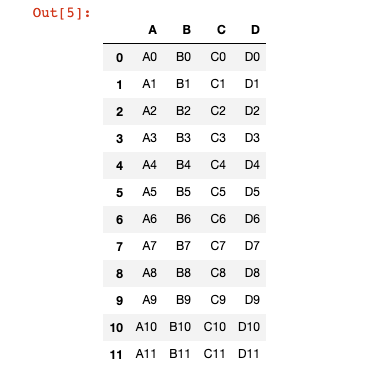# How To Concatenate DataFrames in Pandas

Hey - Nick here! This page is a free excerpt from my \$199 course Python for Finance, which is 50% off for the next 50 students.

In this lesson, we will learn how to concatenate pandas DataFrames. This will be a brief lesson, but it is an important concept nonetheless. Let's dig in!

## The DataFrames We'll Use In This Lesson

To demonstrate how to merge pandas DataFrames, I will be using the following 3 example DataFrames:

``````df1 = pd.DataFrame({'A': ['A0', 'A1', 'A2', 'A3'],

'B': ['B0', 'B1', 'B2', 'B3'],

'C': ['C0', 'C1', 'C2', 'C3'],

'D': ['D0', 'D1', 'D2', 'D3']},

index=[0, 1, 2, 3])

df2 = pd.DataFrame({'A': ['A4', 'A5', 'A6', 'A7'],

'B': ['B4', 'B5', 'B6', 'B7'],

'C': ['C4', 'C5', 'C6', 'C7'],

'D': ['D4', 'D5', 'D6', 'D7']},

index=[4, 5, 6, 7])

df3 = pd.DataFrame({'A': ['A8', 'A9', 'A10', 'A11'],

'B': ['B8', 'B9', 'B10', 'B11'],

'C': ['C8', 'C9', 'C10', 'C11'],

'D': ['D8', 'D9', 'D10', 'D11']},

index=[8, 9, 10, 11])``````

## How To Concatenate Pandas DataFrames

Anyone who has taken my Introduction to Python course will remember that string concatenation means adding one string to the end of another string. An example of string concatenation is below.

``````str1 = "Hello "

str2 = "World!"

str1 + str2

#Returns 'Hello World!'``````

DataFrame concatenation is quite similar. It means adding one DataFrame to the end of another DataFrame.

In order for us to perform string concatenation, we should have two DataFrames with the same columns. An example is below:

``pd.concat([df1, df2, df3])``By default, pandas will concatenate along `axis=0`, which means that its adding rows, not columns.

If you want to add rows, simply pass in `axis=0` as a new variable into the `concat` function.

``pd.concat([df1,df2,df3],axis=1)``

In our case, this creates a very ugly DataFrame with many missing values:## Moving On

That concludes our brief discussion of DataFrame concatenation. Let's dig into some practice problems next!